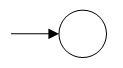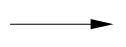# Finite automata

Finite automata can be described into two types.

1: Deterministic Finite Automata (DFA).

2: Non Deterministic Finite Automata (NDFA).

#### Deterministic Finite Automata:

DFA can be described as

• The total number of the alphabet should be finite.
• The total number of states should be finite.
• The total number of transitions should be finite.
• It is required that the number of transition expecting from the states must be equal to the total number of the alphabet.

#### Graphical Representation of DFA:

States:

There are two types of states.

1: Initial State

2: Final State

Initial State:

There must be one initial state of automata. The initial state is the state from which our language starts.

The symbol of Initial State is as follows,Final State:

The final state of DFA can be one or more than one.

The symbol of the final state is as follows,Transition:

The movement from one state to another state is known as transition.

The symbol of transition is as follows,Example

Let a deterministic finite automaton be as follows

• P = [x, y, z],
• ∑ = [0, 1],
• Initial = [x],
• Final = [c], and

Transition function δ as shown by the following table −

 Present State Next State for Input 0 Next State for Input 1 x X y y Z x z Y z

Its graphical representation would be as followsAutomata Theory